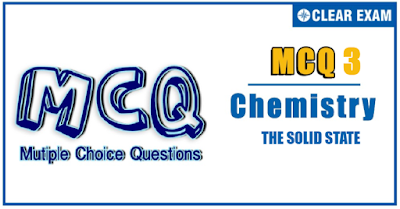## The Solid State Quiz-3

IIT JEE exam which consists of JEE Main and JEE Advanced is one of the most important entrance exams for engineering aspirants. The exam is held for candidates who are aspiring to pursue a career in the field of engineering and technical studies.Chemistry is important because everything you do is chemistry! Even your body is made of chemicals. Chemical reactions occur when you breathe, eat, or just sit there reading. All matter is made of chemicals, so the importance of chemistry is that it's the study of everything..

Q1.Which of the following is not a ferroelectric compound?:
•  Rochelle salt
•  K4[FeCN6]
•  BaTiO3
•  KH2PO4
Solution
(b) Factual statement

Q2.The number of unit cells in 58.5 g of NaCl is nearly:
•  6 × 10^20
•  3 × 10^22
•  1.5 ×10^23
•  0.5×10^24
Solution
(c) 58.5 g NaCl=1 mol =6.02×10^23Na⨁Cl⊝ units One unit cell contains 4 Na⨁Cl⊝ units. Hence, number of the unit cell present = 6.02×10^23/4=1.5×10^23

Q3.  The density of an ionic compound (Mw=58.5) is 2.165 kg m-3 and the edge length of unit cell is 562 pm, then the closest distance between AB⊝ and Zeff of unit cell is
•  281 pm,4
•   562 pm,2
•   562 pm,4
•   281 pm,2
Solution
(a) ρ=Zeff*Mw/a3*NA ∴Zeff=4 (fcc-type structure) ∴For fcc, dA-B⊝=a2=5622=281 pm

Q4. The interionic distance for cesium chloride crystal will be
•  a
•  a/2
•  root(3)a/2
•  2a/root(3)
Solution
(c) As CsCl is body-centered,d=sqrt(3)a/2

Q5.The number of nearest neighbours and next nearest neighbours of an Na ion in a crystal of NaCl are, respectively,
•  6Na,12Cl⊝
•  6Cl⊝,12Na
•  12Cl⊝,12Na
•  6Cl⊝,6Na
Solution
(b) Na lies in OVs formed by Cl⊝ (Na touches six Cl⊝ ions) Na and Cl⊝ are not shown touching in the figure. Each atom shown is present at the face center of each cube Distance between two Na and Cl⊝=a2 Try to visualize two cubes exactly above these two cubes.Each atom shown is present at the edge center and body center of each cube Distance between two nearest Na=a2 Thus, the number of nearest neighbours of Na ion = 6 Cl⊝ ions The number of next nearest neighbours of Na ion = 12 Na ions Note: Next nearest neighbours are shown by number 1, 2, 3,…6 in Fig. b.Likeswise 6 next nearest neighbours of Na ions will be in the above two cube. Hence, total number of next nearest neightbours of Na ions=12 Na ions

Q6. A metal of density 7.5 × 103 kg m-3 has an fcc crystal structure with lattice parameter a = 400 pm. Calculate the number of unit cells present in 0.015 kg of the metal
•  6.250×10^22
•  3.125×10^23
•  3.125×10^22
•  1.563×10^22
Solution
(c) The volume available =0.0157.5×103 (Number of unit cells)400×10-123=0.0157.5×103 Number of unit cell =3.125×10^22

Q7.If R is the radius of the octahedral voids and r is the radius of the atom in close packing, then r/R is equal to
•  2.41
•  4.76
•  3.22
•  9.1
Solution
(a) For OV, R/r i.e., r/r⊝or rvoid/r⊝=0.414 ∴r/R=1/0.414=2.41

Q8.In cubic ZnS (II-VI) compounds, if the radii of Zn and S atoms are 0.74 Å and 1.70 Å, the lattice parameter of cubic ZnS is
•  11.87 Å
•  5.634 Å
•  5.14 Å
•  2.97 Å
Solution
(b) rri.e.,rZnrS2-=0.74 Å1.70 Å=0.44 From radius ratio, it is expected that Zn2+ ion occupy OVs; however, the value of 0.44 is only slightly larger than rvoid/r=0.414 for OV. There is also some covalent character in the Zn2+-S2- interaction, which tends to shorten the interaction distance Note: Experimentally, it was found that Zn2+ ions occupy TVs ∴rZn2++rS2-=34a 0.74+1.70Å=34a⇒a=5.634 Å

Q9.Which of the following is a ferroelectric compound?
•  BaTiO3
•  K4FeCN6
•  Pb2O3
•  None of these
Solution
(a) Factual statement

Q10.Silver (atomic weight =108 g mol-1) has a density of 10.5 g cm-3. The number of silver atoms on a surface of area 10-12m2 can be expressed in scientific notation as y10x. The value of x is
•  3
•  4
•  7
•  8
Solution
(c) Volume of one mole of silver atoms =10810.5cm3/mol Volume of one silver atom =10810.516.022 ×1023 cm3 So, 43r3=10810.516.022 ×1023=1.708×10-23 r3=0.407×10-23cm3=0.407×10-29m3 Area of each silver atom, r2=0.407×10-29 m32/3 So, number of silver atoms in given area =10-120.407×10-29 m32/3=108π×2 =1.6×107=y10x So, x=7#### Written by: AUTHORNAME

AUTHORDESCRIPTION## Want to know more

Please fill in the details below:

## Latest NEET Articles\$type=three\$c=3\$author=hide\$comment=hide\$rm=hide\$date=hide\$snippet=hide

Name

ltr
item
BEST NEET COACHING CENTER | BEST IIT JEE COACHING INSTITUTE | BEST NEET & IIT JEE COACHING: The Solid State Quiz-3
The Solid State Quiz-3
https://1.bp.blogspot.com/-cofWGSXPtMc/YN9ApOquecI/AAAAAAAAGv8/BgbbWVcgt6QGifgz2AI3EiQEo5p-MaBXQCLcBGAsYHQ/w400-h209/MCQ3.PNG
https://1.bp.blogspot.com/-cofWGSXPtMc/YN9ApOquecI/AAAAAAAAGv8/BgbbWVcgt6QGifgz2AI3EiQEo5p-MaBXQCLcBGAsYHQ/s72-w400-c-h209/MCQ3.PNG
BEST NEET COACHING CENTER | BEST IIT JEE COACHING INSTITUTE | BEST NEET & IIT JEE COACHING
https://www.cleariitmedical.com/2021/07/the-solid-state-quiz-3.html
https://www.cleariitmedical.com/
https://www.cleariitmedical.com/
https://www.cleariitmedical.com/2021/07/the-solid-state-quiz-3.html
true
7783647550433378923
UTF-8

STAY CONNECTED